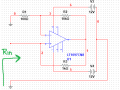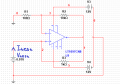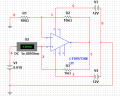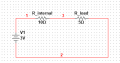# Negative input resistance - OPAMP circuit

#### xxxyyyba

Joined Aug 7, 2012
289
Hi.
I study OPAMP circuit with negative input resistance. Here is schematic:To find Rin, I connect test generator:Rin would be Vtest/Itest.
I get Rin = - R1 * R3 / R2 = -10 Ohm. (for ideal OPAMP)
For real OPAMP (LT1097CN8), input impendance will be very close to ideal one I got:Rin = 0.01V / (-1.001mA) = - 9.99 Ohm.

My question is, how can I use this circuit? For example, If I have this circuit:How should I connect OPAMP circuit to this circuit to cancel source internal resistance R_internal? Current in that case would be:
I = V1 / (R_internal + R_load - Rin) = V1 / R_load.

Last edited:

#### DickCappels

Joined Aug 21, 2008
8,701
•xxxyyyba